# 8天玩转并行开发——第八天 用VS性能向导解剖你的程序

最后一篇，我们来说说vs的“性能向导"，通常我们调试程序的性能一般会使用Stopwatch,如果希望更加系统的了解程序，我们就需要

 1 using System;
2 using System.Collections.Generic;
3 using System.Linq;
4 using System.Text;
5 using System.Diagnostics;
6
7 namespace Test
8 {
9     class Program
10     {
11         static object obj = new object();
12
13         static void Main(string[] args)
14         {
15             var watch = Stopwatch.StartNew();
16
17             var range = ParallelEnumerable.Range(1, 100000000);
18
19             var query = (from n in range.AsParallel()
20                          where n % 5 == 0
21                          select Calculate(n)).Average();
22
23             watch.Stop();
24
25             Debug.WriteLine("耗费时间:{0}", watch.Elapsed);
26             Console.WriteLine("耗费时间:{0}", watch.Elapsed);
27         }
28
29         /// <summary>
30         /// 模拟复杂的数学计算
31         /// </summary>
32         /// <param name="num"></param>
33         /// <returns></returns>
34         static double Calculate(int num)
35         {
36             lock (obj)
37             {
38                 var sqrt = Math.Sqrt(num);
39
40                 var pow = Math.Pow(sqrt, 5);
41
42                 var log10 = Math.Log10(pow);
43
44                 var floor = Math.Floor(log10);
45
46                 return floor;
47             }
48         }
49     }
50 }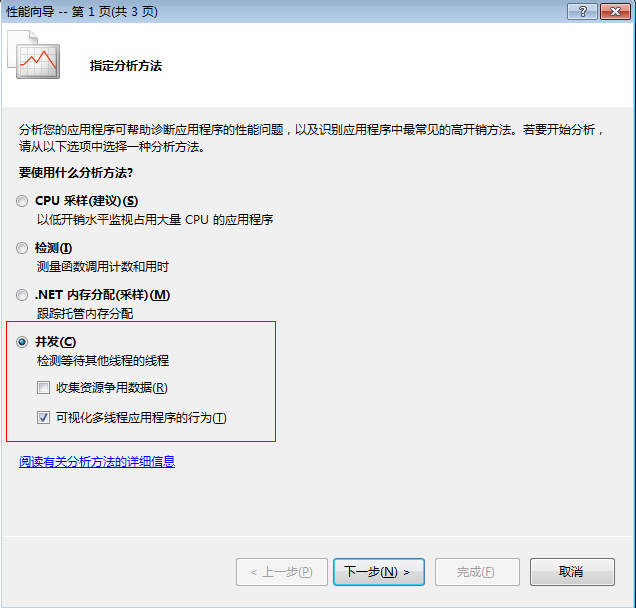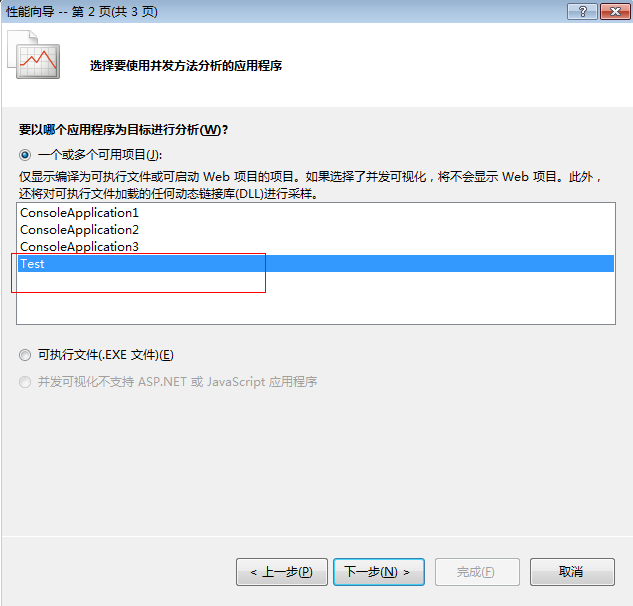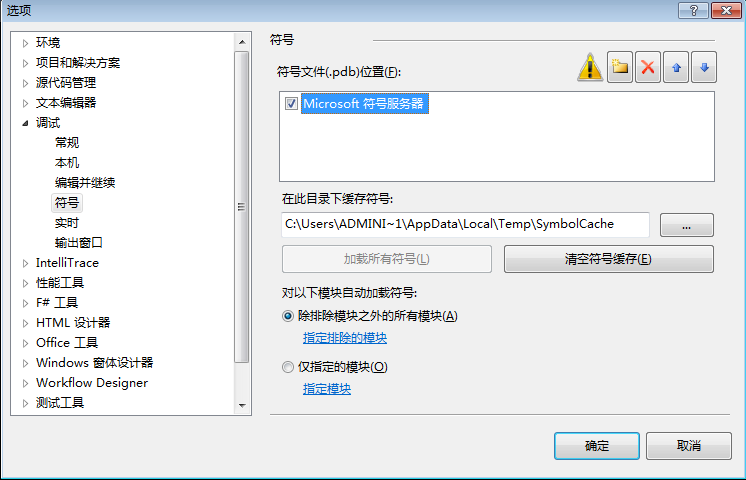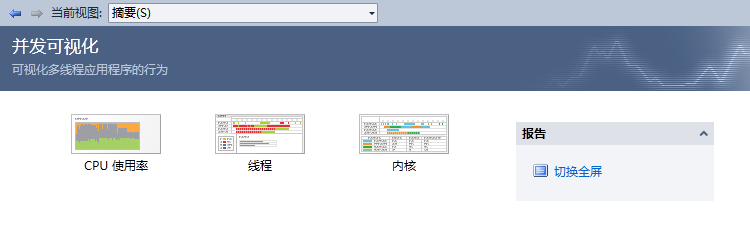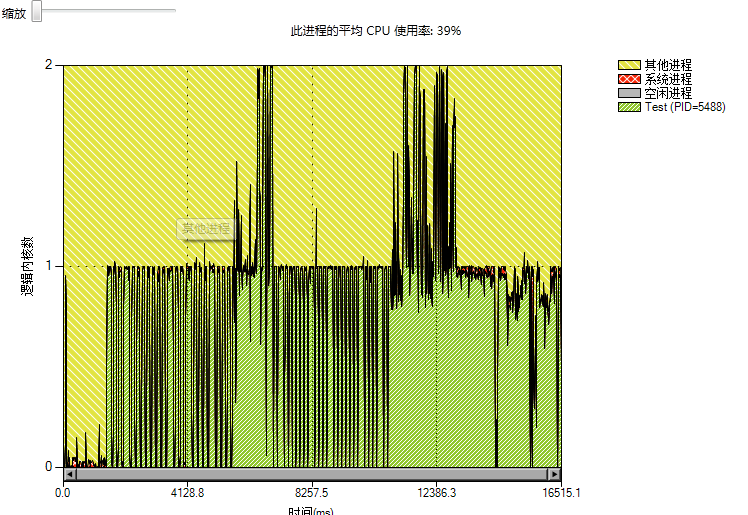①：从图中的绿色破浪线可以看出，我们的程序确实是多核计算。

②：并行计算耗时16515ms,平均CPU使用率：39%。，这里要注意，性能剖析器也需要耗费CPU周期,所以执行时间要稍大于实际时间。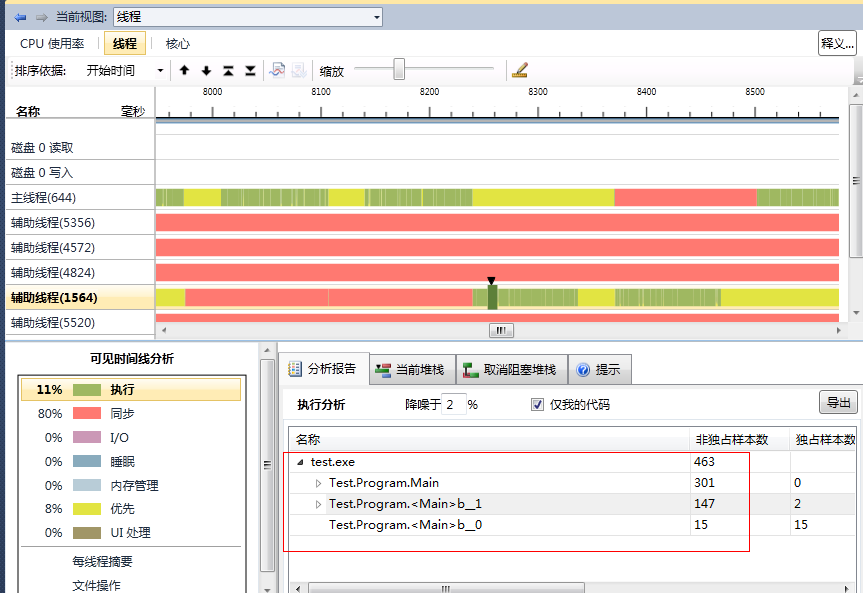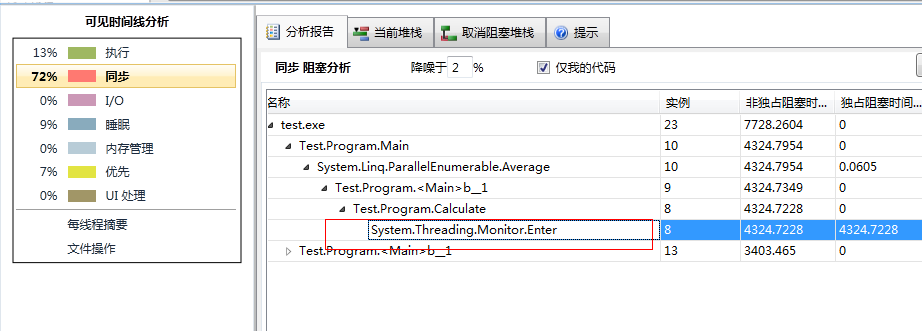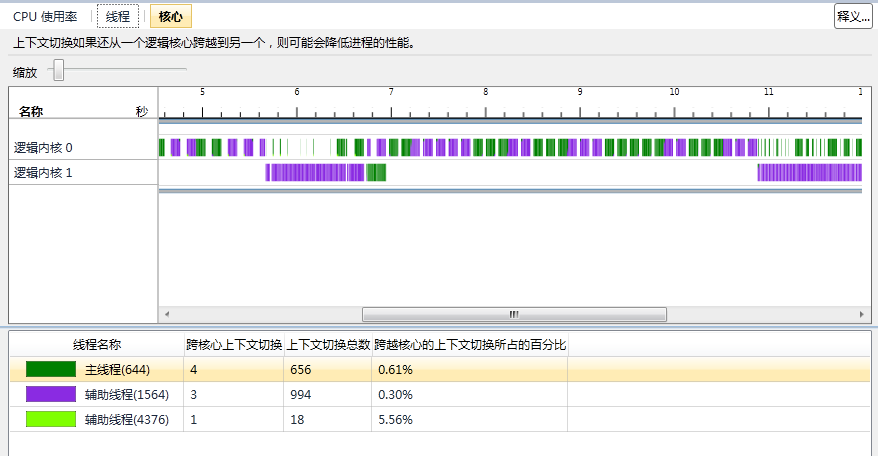1         static double Calculate(int num)
2         {
3                 var sqrt = Math.Sqrt(num);
4
5                 var pow = Math.Pow(sqrt, 5);
6
7                 var log10 = Math.Log10(pow);
8
9                 var floor = Math.Floor(log10);
10
11                 return floor;
12         }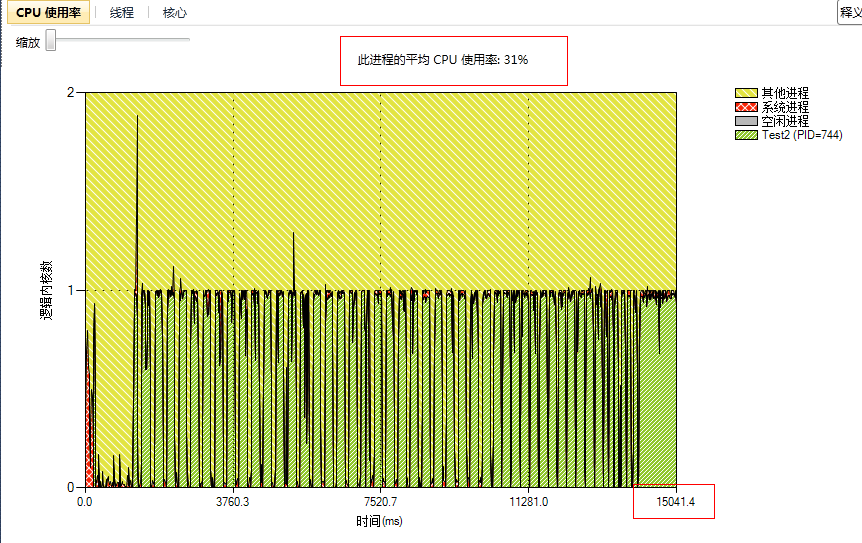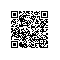使用钉钉扫一扫加入圈子
+ 订阅